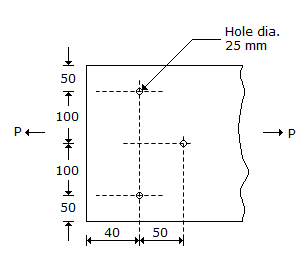# Civil Engineering - UPSC Civil Service Exam Questions

36.

Two pipe systems in series are said to be equivalent when

 A. the average diameter in both systems is same B. the average friction factor in both systems is same C. the total length of the pipes is same in both the systems D. the discharge under the same head is same in both the systems

Explanation:

No answer description available for this question. Let us discuss.

37.

A summit curve is formed at the intersection of a 3% upgrade and a 5% downgrade. What is the length of the summit curve in order to provide a stopping distance of 128 m ?

 A. 271m B. 298 m C. 322 m D. 340 m

Explanation:

No answer description available for this question. Let us discuss.

38.

Two spheres of mass 15 kg and 20 kg, move along a straight line in the same direction with velocities of 20 m/s and 5 m/s, respectively. If the coefficient of restitution is 0.7, then the velocity of the 15 kg mass after collision will be

 A. 5.43 m/s B. 15.93 m/s C. 18.72 m/s D. 16.16 m/s

Explanation:

No answer description available for this question. Let us discuss.

39.

What is the effective net width of plate shown in the given sketch, for carrying tension ?A. 212.5 mm B. 237.5 mm C. 250 mm D. 275 mm

Explanation:

No answer description available for this question. Let us discuss.

40.

In a project, the contractor is paid on the basis of the running bill for a month. The rate contract for concreting is Rs. 1000/m3 of concrete. The consumption of number of cement of bags of weight 50 kg is 527. The mix proportion for the concrete is 1: 1.4 : 2.75 with W/C ratio of 0.52. The approximate billing amount of the month will be

 A. Rs. 5, 27, 000 B. Rs. 1.49, 404 C. Rs. 62, 252 D. Rs. 26, 350